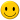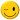## Convert a series of single cell timeseries to a NetCDF file

Hi all!
I have text files with time series (3-hourly) with 6 variables. Each file represents a cell with specific lat-long coordinate.

¿Someone knows how to convert those txt files to one single NetCDF file?

All the txt files represent a grid over Chile, the spatial resolution is 0.05° X 0.05°.

I attach a few files.

Alonso.

### Replies (4)

#### RE: Convert a series of single cell timeseries to a NetCDF file - Added by Karin Meier-Fleischerabout 1 year ago

Hi Alonso,

to convert such an ASCII file where the columns are the 6 different variables you have to write a script to select each variable from the input ASCII file, create a grid description file, and define the starting time.

I've tested it using a ksh script:

```#!/bin/ksh

input=data_-17.475_-69.475

ncols=6                                 #-- number of variables
variables=("var1" "var2" "var3" "var4" "var5" "var6") #-- variable names

time="1950-01-01,00:00:00,3hour"        #-- init time axis

lat=\$(echo \$input | cut -d "_" -f 2)    #-- latitude value
lon=\$(echo \$input | cut -d "_" -f 3)    #-- longitude value

#-- generate the grid description file for \$input
cat << EOF > gridfile_\${input}.txt
gridtype = lonlat
xsize    = 1
ysize    = 1
xvals    = \$lon
yvals    = \$lat
EOF

#-- loop over all variables
j=0
for i in \$(seq 1 \${ncols})
do
echo "--> variable: \${variables[j]}"
\$(cat  \$input | cut -d " " -f \${i} > tmp.txt)
cdo -s -f nc -settaxis,\$time -setname,\${variables[\$j]} -input,gridfile_\${input}.txt out_\$i.nc < tmp.txt
j=\$(expr \$j + 1)
done

#-- merge all variable into one netCDF file
cdo -O -s merge out_*.nc \${input}.nc
```

-Karin

#### RE: Convert a series of single cell timeseries to a NetCDF file - Added by Alonso Arriagadaabout 1 year ago

Hi Karin!

I will try to use your script.

Best!A.

#### RE: Convert a series of single cell timeseries to a NetCDF file - Added by Alonso Arriagadaabout 1 year ago

Hi Karin,
Your script worked fine for a single file, so I have tried to loop over all the files in my folder, but I am not able to run it.
I created a txt file with the names of the files in my forlder called "dirlist.txt"
I have use Cygwyn in Windows10. This is my script:

```#!/bin/mksh

for file in \$(cat  dirlist.txt)
do
input=\$file

ncols=6                                 #-- number of variables
variables=("pr_mm" "WS_m_s" "T_grC" "HR" "SWR_W_m2" "LWR_W_m2") #-- variable names

time="1979-01-01,00:00:00,3hour"        #-- init time axis

lat=\$(echo \$input | cut -d "_" -f 2)    #-- latitude value
lon=\$(echo \$input | cut -d "_" -f 3)    #-- longitude value

#-- generate the grid description file for \$input
cat << EOF > gridfile_\${input}.txt
gridtype = lonlat
xsize    = 1
ysize    = 1
xvals    = \$lon
yvals    = \$lat
EOF

#-- loop over all variables
j=0
for i in \$(seq 1 \${ncols})
do
echo "--> variable: \${variables[j]}"
\$(cat  \$input | cut -d " " -f \${i} > tmp.txt)
cdo -f nc -settaxis,\$time -setname,\${variables[\$j]} -input,gridfile_\${input}.txt out_\$i.nc < tmp.txt
j=\$(expr \$j + 1)
done

#-- merge all variable into one netCDF file
cdo merge out_*.nc \${input}.nc
done
```

Best,

Alonso.

#### RE: Convert a series of single cell timeseries to a NetCDF file - Added by Alonso Arriagadaabout 1 year ago

Hi Karin,
I have solved it! After some time of messing around, I have found the useful script.
I have solve it with a `ls data*.*` in the first for loop. This is the code:

#!/bin/mksh

ncols=6
variables=("pr_mm" "WS_m_s" "T_grC" "HR" "SWR_W_m2" "LWR_W_m2")

time="1979-01-01,00:00:00,3hour"

for var in `ls data_*.*`;
do
input=\$var
echo "\$input"

``lat=\$(echo \$input | cut -d "_" -f 2)    lon=\$(echo \$input | cut -d "_" -f 3)``
``newfile=\${myroot}/gridfile_\${input}.txt    echo "\$newfile"``
``echo "gridtype = lonlat"  > \$newfile    echo "xsize    = 1"      >> \$newfile    echo "ysize    = 1"      >> \$newfile    echo "xvals    = \$lon"   >> \$newfile    echo "yvals    = \$lat"   >> \$newfile``
``j=0    for i in \$(seq 1 \${ncols})    do          echo "j: \$j   i: \$i"           echo "--> variable: \${variables[j]}"           \$(cat  \$input | cut -d " " -f \${i} > tmp.txt)          cdo -s -f nc -settaxis,\$time -setname,\${variables[\$j]} -input,\$newfile out_\$i.nc < tmp.txt          j=\$(expr \$j + 1)    done``
``cdo -s merge out_*.nc \${input}.nc``

done

Thank you! This would not have been possible without your initial help.Best Regards.
Alonso.

(1-4/4)